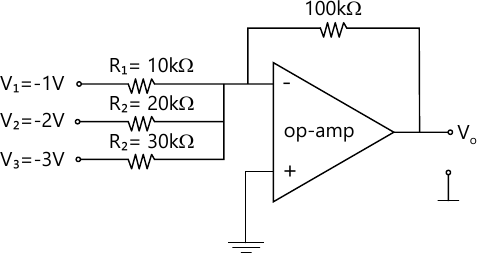MORE IN Basic Electronics
VTU First Year Engineering (C Cycle) (Semester 2)
Basic Electronics
December 2015
Total marks: --
Total time: --
INSTRUCTIONS
(1) Assume appropriate data and state your reasons
(2) Marks are given to the right of every question
(3) Draw neat diagrams wherever necessary

1 (a) Draw and explain the V-I characteristics of a silicon diode.
8 M
1 (b) What is a rectifier? With a neat circuit diagram and waveforms, explain the working of full wave rectifier.
8 M
1 (c) A full wave rectifier with a load of 1 kΩ. The ac voltage applied to the diode is 200-0-200 V, if diode resistance is neglected. Calculate:
i) Average dc current;
ii) Average dc voltage.
4 M

2 (a) Draw and explain the input and output characteristics of common emitter configuration.
8 M
2 (c) Explain full wave rectifier with capacitor filter with necessary waveforms.
7 M

3 (a) With a neat circuit diagram, explain the voltage divider bias circuit by giving its exact analysis.
8 M
3 (b) For the base bias circuit for npn transistor, find IB, IC and VCE if RC=2.2 KΩ, RB=470 KΩ. VCC=18 V, hfe=100. Draw the dc load line and Q point.
8 M
3 (c) What is op-amp? List the ideal characteristics of an op-amp.
4 M

4 (a) Define for an op-amp (i) CMRR, (ii) Slew rate, (iii) PSRR.
6 M
4 (b) Show how an op-amp can be used as integrator. Derive an expression for its output.
6 M
4 (c) For the circuit shown in Fig. Q4(c). Calculate the output voltage.4 M
4 (d) What is voltage follower? Explain.
4 M

5 (a) Convert:
i) (35.45)10 =( )2.
ii) (475.25)8=( )10
iii) (3FD)16 = ( )2.
6 M
5 (b) State and prove DeMorgan's theorems.
6 M
5 (c) Show that: [ i) Aoverline{B}C + B + Boverline{D}+ ABoverline{D} + overline{A}C = B+C \ ii) overline{overline{AB}+overline{A}+AB}=0 \ iii) AB+A(B+C)+B(B+C)=B+AC ]
6 M
5 (d) What are universal gates?
2 M

6 (a) Realize two input EX-OR gate using only NAND gates.
5 M
6 (b) Design full adder and implement it.
7 M
6 (c) Subtract (111001)2 from (101011)2 using 2's complement method.
4 M
6 (d) Realize OR gate using diodes and explain.
4 M

7 (a) Define flip flop. Explain R-S flip flop.
5 M
7 (b) With neat block diagrams, explain architecture of 8085 microprocessor.
10 M
7 (c) List the difference between microprocessor and microcontroller.
5 M

8 (a) What is transducer? Distinguish between active and passive transducer.
5 M
8 (b) With a neat sketch, explain construction and working of LVDT.
7 M
8 (c) Explain the working of photo voltaic transducer.
8 M

9 (a) What is modulation? What is the need of modulation?
5 M
9 (b) A 500, W, 1MHz carrier is amplitude modulated with a sinusoidal signal of 1 kHz. The depth of modulation is 60%. Calculate the bandwidth, power in the sidebands and the total power transmitted.
7 M
9 (c) Define AM. Draw the AM signal and its spectrum. Derive the necessary expression for AM.
8 M

10 (a) With a block diagram, explain typical cellular mobile unit.
5 M
10 (b) What is ISDN? Explain services of ISDN.
6 M
10 (c) Explain advantages and applications of optical fibres.
5 M
10 (d) Give the comparison between AM and FM.
4 M

More question papers from Basic Electronics